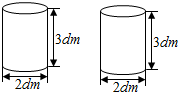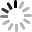4．现有2根圆往形木材（如图），李师傅把其中一根加工成了一个最大的长方体，把另一根加工成了一个最大的圆锥．加工成的长方体和圆锥谁的体积大？大多少？

2×（2÷2）÷2×2×3
=2×1÷2×2×3
=2×3
=6（立方分米）；

$\frac{1}{3}×$3.14×（2÷2）2×3
=$\frac{1}{3}×3.14×1×3$
=3.14（立方分米），
6-3.14=2.86（立方分米）；